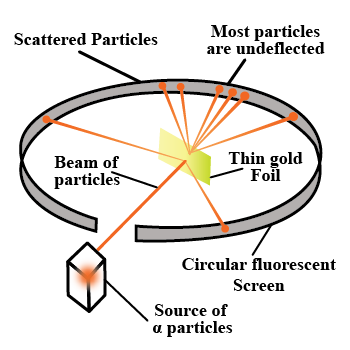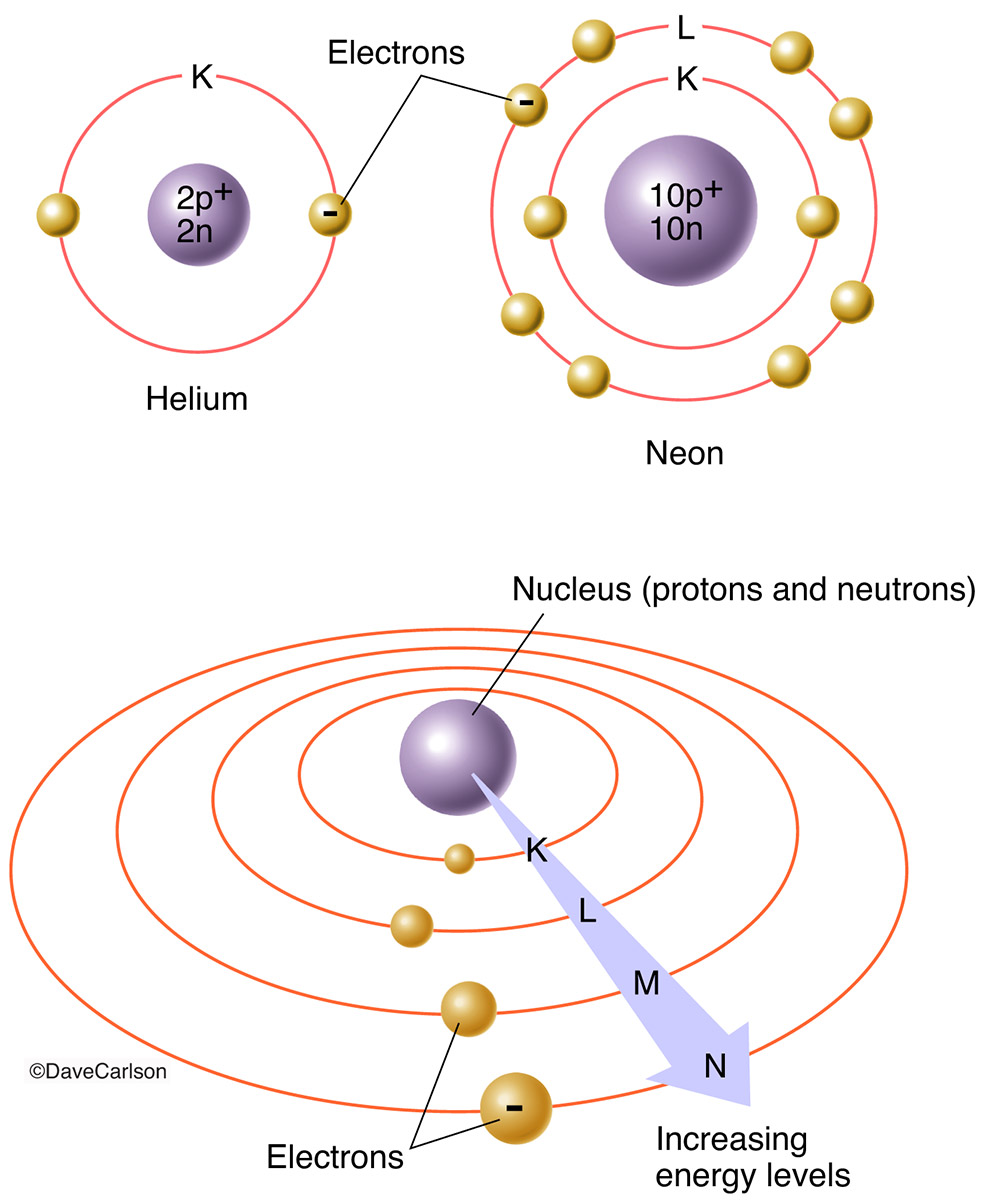# CBSE Class 12 Physics Chapter 12 Revision Notes

Chapter 12: Atoms Revision Notes

• Every element is made up of tiny, invisible particles known as atoms.
• Every atom is a sphere with a radius of 10-10 m in which the entire mass is uniformly distributed and the nucleus is surrounded by negatively charged electrons.
• Experimental setup for the ɑ-scattering experiment and the path taken by the ɑ-particlesSource

• The impact parameter is the perpendicular distance between the velocity vector of a particle and the central line of the nucleus of the atom.

## Basic Assumptions of Rutherford’s Atomic Model

• Atoms are made up of a small central core known as the atomic nucleus, which is thought to contain all of the mass and positive charge.

• The nucleus is a fraction of the size of the atom.

• Electrons surround the nucleus, making the atom electrically neutral.

• The nucleus is surrounded by electrons, and the centripetal force is electrostatic.

• Distance of Closest Approach:

The KE of a ɑ-particle converts to electrostatic potential energy at a distance r0 from the nucleus, and the -particle can no longer approach the nucleus; this distance (r0) is known as the distance of closest approach.

• Angle of Scattering:

Angle of scattering is the angle by which a particle deviates from its original path around the nucleus.

• Rutherford’s Model has a number of flaws

(i) I was unable to clearly explain the stability of an atom.

(ii) Line spectrum cannot be explained.

## Bohr’s Theory of the Hydrogen Atom

• Bohr’s theory was composed of three postulates that combined classical and early quantum concepts. These are
• Bohr’s first postulate, which stated that an electron in an atom could revolve in certain stable orbits without emitting radiant energy, despite electromagnetic theory’s predictions.
• These stable orbits are defined by Bohr’s second postulate. According to this postulate, the electron revolves around the nucleus only in orbits where the angular momentum is an integral multiple of h/2π, where h is Planck’s constant (= 6.6 x 10-34 J – s). As a result, the orbiting electron’s angular momentum (L) is quantized, i.e. L = nh/2π. Because the electron’s angular momentum (L) = mvr, mvr = nh/2π for any permitted (stationary) orbit

where n is any positive integer, such as 1, 2, 3, and so on.

It’s also referred to as the principal quantum number.

• According to Bohr’s third postulate, an electron may transition from one of its non-radiating orbits to a lower-energy orbit. When this happens, a photon with the same energy as the energy difference between the initial and final states is emitted.

hv – Ei– Ef gives the frequency of the emitted photon, where Ei and Ef are the energies of the initial and final states, respectively, and Ei > Ef.

• Bohr’s Model Limitations

(i) Only applies to hydrogen-like atoms.

(ii) Does not account for the fine structure of H-atom spectral lines.

(iii) The shape of the orbit is not explained.

• Energy Level:Source

• When an atom’s electron is revolving in the orbit closest to the nucleus, n = 1, the energy of the atom is the lowest.

• The ground state of an atom is the lowest and most energy-dense state of the atom. This state has an energy of -13.6 eV. As a result, the minimum energy required to free an electron from the hydrogen atom’s ground state is -13.6 eV.

• Emission Spectrum: The hydrogen spectrum, also known as the hydrogen emission spectrum, is made up of discrete bright lines on a dark background.

• Absorption Spectrum: Another type of hydrogen spectrum exists in which dark lines appear on a bright background; this is known as an absorption spectrum.

]]>